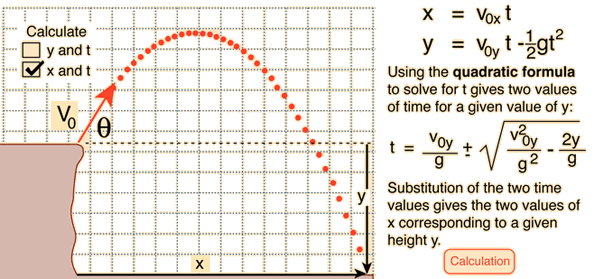# A soccer ball leaves a cliff 20.2 m above the valley ﬂoor, at an angle of 10 degrees above the horizontal. The ball hits the valley floor 3.0 seconds later. What is the initial velocity of the ball?

Dec 2, 2015

$46.05 \text{m/s}$

#### Explanation:

The situation looks like this:I will adopt a convention that "up is positive". This means that $y$ will be -ve in this scenario.

We can use:

$s = u t + \frac{1}{2} a {t}^{2}$

This becomes:

$y = v \left(\sin \theta\right) t - \frac{1}{2} \text{g} {t}^{2}$

$\therefore - 20.2 = 3 v \sin \theta - \frac{1}{2} \times 9.8 \times {3}^{2}$

$\therefore 3 v \sin \theta = 44.1 - 20.2 = 23.9$

$\therefore v = \frac{23.9}{3 \sin 10}$

$v = \frac{23.9}{3 \times 0.173} = 46.05 \text{m/s}$